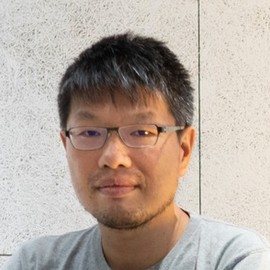## Te-Sheng Lin (林得勝)

### National Yang Ming Chiao Tung University, TW

I am an associate professor at the Department of Applied Mathematics at National Yang Ming Chiao Tung University, Taiwan. My research focuses on developing analytical and computational tools and further communicating with scientists from other disciplines to solve practical engineering problems.

I received my Ph.D. degree in Applied Mathematics from the Department of Mathematical Sciences at New Jersey Institute of Technology, where I worked with Lou Kondic and Linda J. Cummings.

My list of publications in Google Scholar. Some preprints in arXiv.

I co-organize NYCU webinar on Scientific Machine Learning.

### Interests

• Mathematical modeling
• Scientific computation
• Scientific machine learning

### Education

• PhD in Applied Mathematics, 2012

New Jersey Institute of Technology, USA

• M.S. in Applied Mathematics, 2004

National Chung Cheng University, Taiwan

• B.S. in Mathematics, 2002

National Chung Cheng University, Taiwan

# Appointments

#### National Yang Ming Chiao Tung University

Feb 2021 – Present Hsinchu, TW

#### National Chiao Tung University

Aug 2020 – Jan 2021 Hsinchu, TW

#### National Chiao Tung University

Aug 2014 – Jul 2020 Hsinchu, TW

#### Loughborough University

Dec 2012 – Jul 2014 Loughborough, UK

#### Loughborough University

Jun 2012 – Dec 2012 Loughborough, UK

# Recent Publications

(2023). A cusp-capturing PINN for elliptic interface problems. J. Comput. Phys..

(2023). Analysis and classification of coffee beans using single coffee bean mass spectrometry with machine learning strategy. Jood Chem..

(2023). An efficient neural-network and finite-difference hybrid method for elliptic interface problems with applications. Comm. Comput. Phys..

(2023). On the transition to dripping of an inverted liquid film. J. Fluid Mech..

(2022). A Reduced Model for a Phoretic Swimmer. J. Fluid Mech..

(2022). A Discontinuity Capturing Shallow Neural Network for Elliptic Interface Problems. J. Comput. Phys..

(2022). A Shallow Ritz Method for elliptic problems with Singular Sources. J. Comput. Phys..

(2022). Spontaneous locomotion of phoretic particles in three dimensions. Phys. Rev. Fluids.

(2021). Thin liquid films in a funnel. J. Fluid Mech..

# Recent Posts

### Euler equations

Euler equations Here, we consider Euler equations: $$\tag{1} x^2 y’’ + a xy’ + by = 0, \quad x\ne 0,$$ where $a$, …

### Euler equations

Euler equations 這裡我們想要解 Euler equations, 長相如下: $$\tag{1} x^2 y’’ + a xy’ + by = 0,$$ 其中 $a$, $b$ 為常數. …

### Power method with Rayleigh Quotient

Power 迭代法目錄: 基本概念 Power iteration; inverse power method; shifted inver power method 找第二大的 eigenvalue deflation Rayleigh Quotient 迭代及其收斂 …

### Power method - deflation

Power 迭代法目錄: 基本概念 Power iteration; inverse power method; shifted inver power method 找第二大的 eigenvalue deflation Rayleigh Quotient 迭代及其收斂 …

### Power method

Power 迭代法目錄: 基本概念 Power iteration; inverse power method; shifted inver power method 找第二大的 eigenvalue deflation Rayleigh Quotient 迭代及其收斂 …